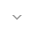# MathContext

`public final class MathContext`
`extends Object` `implements Serializable`

 java.lang.Object ↳ android.icu.math.MathContext

`MathContext`不可变类封装了`BigDecimal`类（以及可能的其他类）的运算符方法所理解的设置。 运算符方法是那些对数字或一对数字进行操作的方法。

1. `digits`: the number of digits (precision) to be used for an operation
2. `form`: the form of any exponent that results from the operation
3. `lostDigits`: whether checking for lost digits is enabled
4. `roundingMode`: the algorithm to be used for rounding.

`MathContext.DEFAULT`提供了一种用于 `MathContext`参数，则使用默认设置（ `9, SCIENTIFIC, false, ROUND_HALF_UP` ）。

`BigDecimal`类中，接受`MathContext`对象的所有方法`MathContext`默认值）也具有不接受MathContext参数的方法版本。 这些版本执行无限精度的定点算术（就像设置为（ `0, PLAIN, false, ROUND_HALF_UP` ））。

• `BigDecimal`

## Summary

### Constants

`int` `ENGINEERING`

`int` `PLAIN`

`int` `ROUND_CEILING`

`int` `ROUND_DOWN`

`int` `ROUND_FLOOR`

`int` `ROUND_HALF_DOWN`

`int` `ROUND_HALF_EVEN`

`int` `ROUND_HALF_UP`

`int` `ROUND_UNNECESSARY`

`int` `ROUND_UP`

`int` `SCIENTIFIC`

### Fields

` public static final MathContext` `DEFAULT`

### Public constructors

` MathContext(int setdigits) `

` MathContext(int setdigits, int setform) `

` MathContext(int setdigits, int setform, boolean setlostdigits) `

` MathContext(int setdigits, int setform, boolean setlostdigits, int setroundingmode) `

### Public methods

` int` ` getDigits() `

` int` ` getForm() `

` boolean` ` getLostDigits() `

` int` ` getRoundingMode() `

` String` ` toString() `

### Inherited methodsFrom class ` java.lang.Object `

## Constants

### ENGINEERING

`int ENGINEERING`

• `PLAIN`
• `SCIENTIFIC`

### PLAIN

`int PLAIN`

• `ENGINEERING`
• `SCIENTIFIC`

### ROUND_CEILING

`int ROUND_CEILING`

### ROUND_DOWN

`int ROUND_DOWN`

### ROUND_FLOOR

`int ROUND_FLOOR`

### ROUND_HALF_DOWN

`int ROUND_HALF_DOWN`

### ROUND_HALF_EVEN

`int ROUND_HALF_EVEN`

### ROUND_HALF_UP

`int ROUND_HALF_UP`

### ROUND_UNNECESSARY

`int ROUND_UNNECESSARY`

### ROUND_UP

`int ROUND_UP`

### SCIENTIFIC

`int SCIENTIFIC`

• `ENGINEERING`
• `PLAIN`

## Fields

### DEFAULT

`MathContext DEFAULT`

• `SCIENTIFIC`
• `ROUND_HALF_UP`

## Public constructors

### MathContext

`MathContext (int setdigits)`

Parameters
`setdigits` `int`: The `int` digits setting for this `MathContext`.
Throws
`IllegalArgumentException` parameter out of range.

### MathContext

```MathContext (int setdigits,
int setform)```

Parameters
`setdigits` `int`: The `int` digits setting for this `MathContext`.
`setform` `int`: The `int` form setting for this `MathContext`.
Throws
`IllegalArgumentException` parameter out of range.

### MathContext

```MathContext (int setdigits,
int setform,
boolean setlostdigits)```

Parameters
`setdigits` `int`: The `int` digits setting for this `MathContext`.
`setform` `int`: The `int` form setting for this `MathContext`.
`setlostdigits` `boolean`: The `boolean` lostDigits setting for this `MathContext`.
Throws
`IllegalArgumentException` parameter out of range.

### MathContext

```MathContext (int setdigits,
int setform,
boolean setlostdigits,
int setroundingmode)```

Parameters
`setdigits` `int`: The `int` digits setting for this `MathContext`.
`setform` `int`: The `int` form setting for this `MathContext`.
`setlostdigits` `boolean`: The `boolean` lostDigits setting for this `MathContext`.
`setroundingmode` `int`: The `int` roundingMode setting for this `MathContext`.
Throws
`IllegalArgumentException` parameter out of range.

## Public methods

### getDigits

`int getDigits ()`

Returns
`int` an `int` which is the value of the digits setting

### getForm

`int getForm ()`

Returns
`int` an `int` which is the value of the form setting

### getLostDigits

`boolean getLostDigits ()`

Returns
`boolean` a `boolean` which is the value of the lostDigits setting

### getRoundingMode

`int getRoundingMode ()`

Returns
`int` an `int` which is the value of the roundingMode setting

### toString

`String toString ()`

1. `digits=`, immediately followed by the value of the digits setting as a numeric word.
2. `form=`, immediately followed by the value of the form setting as an uppercase word (one of `SCIENTIFIC`, `PLAIN`, or `ENGINEERING`).
3. `lostDigits=`, immediately followed by the value of the lostDigits setting (`1` if enabled, `0` if disabled).
4. `roundingMode=`, immediately followed by the value of the roundingMode setting as a word. This word will be the same as the name of the corresponding public constant.

`digits=9 form=SCIENTIFIC lostDigits=0 roundingMode=ROUND_HALF_UP`

Returns
`String` a `String` representing the context settings.